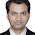## Friday, September 21, 2018

### Coordinating Variable Signs

Someone asked me today (or yesterday, depending on whose time zone you go by) how to force a group of variables in an optimization model to take the same sign (all nonpositive or all nonnegative). Assuming that all the variables are bounded, you just need one new binary variable and a few constraints.

Assume that the variables in question are $x_1,\dots,x_n$ and that they are all bounded, say $L_i \le x_i \le U_i$ for $i=1,\dots,n$. If we are going to allow variables to be either positive or negative, then clearly we need $L_i < 0 < U_i$. We introduce a new binary variable $y$ and, for each $i$, the constraints$$L_i (1-y) \le x_i \le U_i y.$$If $y$ takes the value 0, every original variable must be between its lower bound and 0 (so nonpositive). If $y$ takes the value 1, every original variable must be between 0 and its upper bound (so nonnegative).

Note that trying to enforce strictly positive or strictly negative rather than nonnegative or nonpositive is problematic, since optimization models abhor strict inequalities. The only work around I know is to change "strictly positive" to "greater than or equal to $\epsilon$" for some strictly positive $\epsilon$, which creates holes in the domains of the variables (making values between 0 and $\epsilon$ infeasible).

#### 7 comments:

1.Hi Paul, I applied this constraint in my problem as follows;
------------------
P_max = a; % a is any constant
P_min = -P_max;
cvx_begin
cvx_solver MOSEK
%
variable P(T,U);
variable b(T,1) binary;
%
minimize (obj fn);
%
subject to

P_min .*(1-b) <= P <= P_max .*b;
%
cvx_end
--------------------------------
When I solve this I get "matrix dimension must agree" error.
Any suggestions?

Thanks,
Mohan

1.Sorry, I use neither cvx nor MOSEK. You should try asking on their forums. It might be one of them does not understand chained inequalities.

2.Thanks for reply. The repmat(A,n) command of MATLAB solved the issue.

2.Hi Paul,
I want to enforce following two constraints on two continuous bounded variables X and Y: (1. say limits are same for both or 2. limits are different)
-----------------
Constraint1
If X<0
then Y<=0
---------------------
Constraint2
If X>0
then Y>=0
How can we do it using a binary-variable and upper lower limit technique?
Thanks,
Mohan

1.You have not said what happens to Y if X is zero. If you don't care which sign Y has when X is 0, then the solution above works just fine.

3.Yes, I do not care about Y value when X is zero. What I want is; Y should be "non-positive" when X is "strictly negative", and When X is "strictly positive" then why should take "non-negative values". You mentioned about strictly negative and strictly positive constraints at the end but did not quit get it. Can you please elaborate it a little bit more.

1.The comment about strict inequality had to do with the consequent, not the antecedent. In other words, "if then " works fine using what I posted. On the other hand, "if then " is trickier because math programming models generally do not tolerate strict inequalities. That limitation does not appear relevant to your case.

Due to recent spamming, comments are being moderated. If this is your first time commenting on the blog, please read the Ground Rules for Comments. In particular, if you want to ask an operations research-related question not relevant to this post, consider asking it on Mathematics Stack Exchange.